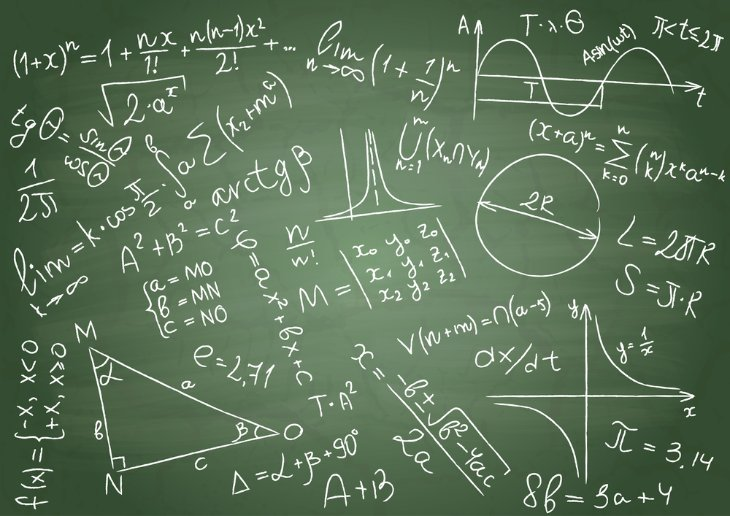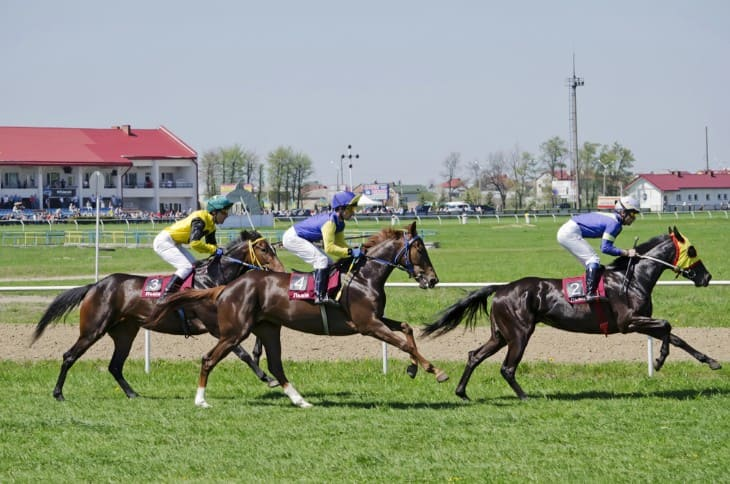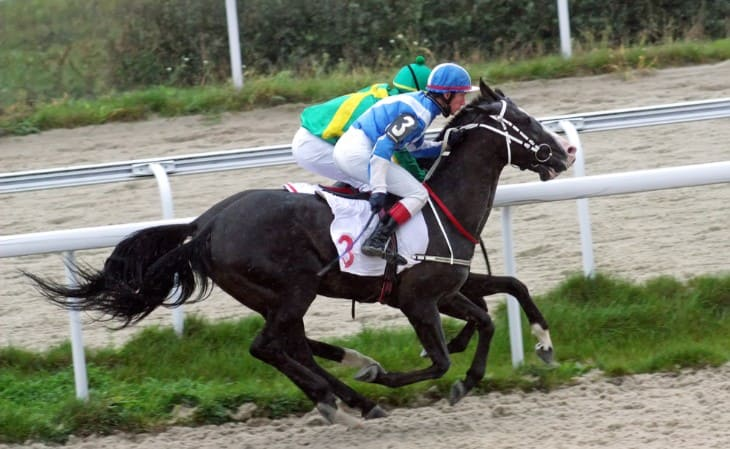The intersection of horse racing and mathematics is a fascinating area that involves applying mathematical concepts and techniques to analyze and improve various aspects of horse racing. Math can play a significant role in understanding the sport, making informed decisions, and potentially increasing the chances of success for bettors, trainers, and owners.

It's important to note that while mathematics can provide valuable insights and tools, horse racing is still a complex and unpredictable sport. Successful application of mathematical techniques requires a deep understanding of both the mathematical principles and the nuances of the racing industry. Additionally, gambling and betting should be approached responsibly, and any strategies developed should be used with caution.

Anyway, let’s take a look at some ways in which mathematics and horse racing intersect:

## Handicapping and Probability

Handicapping and probability are fundamental aspects of horse racing that involve using mathematical analysis to assess the likelihood of a horse's success in a race. Handicapping is the process of evaluating various factors that can influence a horse's performance and using that information to predict its chances of winning or placing well in a race. Probability, on the other hand, involves quantifying these chances in numerical terms.

### Handicapping Factors:

Handicappers consider a wide range of factors when assessing a horse's potential performance. These factors can include:

• Past Performances: Analyzing a horse's previous races, including finishing positions, times, and speed figures.
• Jockey and Trainer Skill: Evaluating the capabilities of the jockey and trainer, as their expertise can significantly impact a horse's performance.
• Class Levels: Assessing the level of competition a horse has faced in previous races and how it compares to the current race.
• Track Conditions: Analyzing how a horse has performed on similar track surfaces and under similar weather conditions.
• Distance and Surface Preferences: Determining whether a horse excels at certain race distances or track surfaces.
• Workouts and Fitness: Examining recent workouts and physical condition to gauge a horse's fitness and readiness.

### Probability and Odds:

Probability is a mathematical concept used to quantify the likelihood of an event occurring. In horse racing, this translates to estimating the probability of a horse winning or finishing in a certain position. Probabilities are often expressed as odds, which represent the potential payout relative to the initial wager. The lower the odds, the higher the perceived probability of the horse winning.

For example, if a horse has odds of 3:1, it is expected to win the race about one out of every four times, based on the bookmakers' assessment. In mathematical terms, the probability of the horse winning would be calculated as:

Probability of Winning = 1 / (1 + Odds)

### Using Mathematics to Make Informed Bets:

Handicappers use mathematics to analyze the handicapping factors and calculate the probabilities of various outcomes. They then compare these calculated probabilities to the odds offered by bookmakers to identify potential value bets. A value bet occurs when the calculated probability is higher than the implied probability of the odds.

Mathematical techniques, such as regression analysis, Bayesian probability, and historical data analysis, can be applied to refine handicapping methods and improve the accuracy of predictions.## Speed Figures and Time Analysis

Speed figures and time analysis are critical tools in the world of horse racing that allows bettors, handicappers, and horsemen to assess a horse's performance based on the time it takes to complete a race. These concepts help quantify a horse's speed and provide a standardized method for comparing performances across different races and tracks. Here's a deeper dive into speed figures and time analysis:

### Speed Figures:

Speed figures are numerical representations of a horse's performance in a race, specifically focusing on the time it takes the horse to complete the distance. They provide a way to compare horses' performances on different tracks and under varying conditions. Speed figures are typically generated by specialized organizations that analyze race results, track conditions, and other relevant factors.

The process of calculating speed figures involves several steps:

Raw Time: The actual time it takes a horse to complete the race distance is recorded.

• Track Variant: Adjustments are made for track conditions on race day. A "fast" track is the standard by which other track conditions are measured.
• Distance Adjustment: Longer or shorter distances can impact a horse's performance, so adjustments are made to account for differences in race distances.
• Weight Carried: The weight carried by a horse can affect its speed, so adjustments are made to factor in the weight each horse carried in the race.
• Class Adjustment: Races of different classes may have different levels of competition, so adjustments are made to account for the class level of the race.

### Time Analysis:

Time analysis involves studying historical race times to identify trends, patterns, and potential insights into a horse's performance. This analysis can include:

• Comparative Analysis: Comparing a horse's race times with those of other horses in the same race or with similar past performances to assess its competitive position.
• Track Records: Evaluating how a horse's time compares to the track record for the same distance and surface.
• Track Biases: Identifying track biases, which are consistent deviations from expected race times due to track conditions. For example, a track may favour front-runners or closers under certain conditions.
• Consistency: Assessing a horse's consistency by analyzing its race times over multiple races to identify any improvements or declines in performance.

### Using Speed Figures and Time Analysis:

Speed figures and time analysis provide valuable information for handicappers and bettors. They can help identify horses that have consistently performed well in terms of speed, regardless of the specific conditions of a particular race. Bettors can use speed figures to compare horses' abilities and assess their chances in upcoming races. Trainers and horsemen can use time analysis to track the progress of their horses, adjust training regimens, and make strategic decisions about race placement.

## Regression Analysis

Regression analysis is a statistical technique used in various fields, including horse racing, to model the relationship between one or more independent variables (also known as predictors) and a dependent variable (the outcome or response variable). In the context of horse racing, regression analysis can be applied to analyze the impact of different factors on a horse's performance, such as finishing position, speed, or odds of winning.

Here's a deeper look into how regression analysis is used in horse racing:

### Types of Regression Analysis:

There are different types of regression analysis that can be applied to horse racing data:

• Linear Regression: Linear regression models the relationship between the independent variables and the dependent variable using a straight line. It is used when there is a linear relationship between the variables. For example, you could use linear regression to model the relationship between a horse's past performance (independent variable) and its finishing position in a race (dependent variable).
• Multiple Regression: Multiple regression involves more than one independent variable. It allows you to analyze the impact of multiple factors on the dependent variable simultaneously. For instance, you could use multiple regression to assess how a combination of jockey skill, track conditions, and recent workouts collectively affect a horse's speed figure.
• Logistic Regression: Logistic regression is used when the dependent variable is binary (two possible outcomes), such as whether a horse wins a race or not. It models the probability of the binary outcome based on the independent variables. Logistic regression could be used to predict the likelihood of a horse winning a race based on its previous performance and other factors.

### Steps in Regression Analysis:

The process of conducting regression analysis involves several steps:

• Data Collection: Gather relevant data on the independent and dependent variables for a set of races or horses.
• Data Preparation: Clean and preprocess the data, handling missing values and outliers.
• Model Building: Choose the appropriate type of regression analysis based on the nature of the data and the research question. Specify the independent variables and build the regression model.
• Model Evaluation: Assess the goodness of fit of the model to the data. This involves evaluating metrics such as the coefficient of determination (R-squared), p-values, and other relevant statistics.
• Interpretation: Interpret the coefficients of the regression model to understand the relationship between the independent variables and the dependent variable. For example, a positive coefficient for a specific factor indicates that an increase in that factor is associated with an increase in the dependent variable.
• Prediction and Inference: Use the regression model to make predictions about future races or draw inferences about the impact of different factors on a horse's performance.

### Applications in Horse Racing:

Regression analysis in horse racing can be applied to various scenarios, such as:

• Analyzing how different factors (past performance, jockey skill, track conditions, etc.) contribute to a horse's finishing position or speed.
• Predicting a horse's odds of winning a race based on its recent form and other relevant factors.
• Investigating the relationship between a horse's performance and its breeding, training regimen, or race distance preferences.
• Identifying track biases and their influence on race outcomes.

### Limitations and Considerations:

While regression analysis can provide valuable insights into the relationships between variables, it's important to recognize its limitations. Correlation does not imply causation and other unaccounted-for factors could influence race outcomes. Additionally, the quality and accuracy of the regression model depend on the quality of the data and the appropriateness of the chosen model.

In summary, regression analysis is a powerful tool in horse racing that enables the exploration of relationships between variables and the prediction of outcomes. When used alongside other handicapping techniques, regression analysis can contribute to more informed decisions by providing a quantitative understanding of how different factors impact a horse's performance.

## Monte Carlo Simulations

Monte Carlo simulations are a computational technique used to model and analyze complex systems or processes by generating a large number of random samples to simulate various possible outcomes. In the context of horse racing, Monte Carlo simulations can be applied to predict race outcomes, assess betting strategies, and analyze the potential impact of different factors on a horse's performance. Here's a deeper dive into how Monte Carlo simulations work and their applications in horse racing:

### How Monte Carlo Simulations Work:

Monte Carlo simulations involve the following steps:

• Define Parameters: Identify the variables and factors that influence the system you want to model. For horse racing, these variables could include horse performance metrics, jockey skills, track conditions, and more.
• Generate Random Samples: Create a large number of random samples (iterations) for each variable within its defined range. These random samples represent different possible scenarios or outcomes.
• Model the System: Apply the defined rules and relationships to each set of random samples to simulate the system's behaviour. In horse racing, this could involve simulating races based on random samples of horse performance and other factors.
• Analyze Results: Collect data from the simulations and analyze the distribution of outcomes. This could include calculating probabilities, averages, variances, and other relevant statistics.
• Draw Conclusions: Draw conclusions and make predictions based on the analysis of the simulation results. These conclusions can help you better understand the potential range of outcomes and assess the likelihood of different events.

### Applications in Horse Racing:

Monte Carlo simulations have various applications in horse racing:

• Race Outcome Prediction: Simulate races using random samples of horse performance, track conditions, and other factors to estimate the probability of each horse winning, placing, or showing.
• Betting Strategy Analysis: Assess different betting strategies by simulating numerous races and tracking the success of each strategy over time. This can help bettors determine optimal betting amounts and approaches.
• Track Bias Analysis: Simulate races with varying track bias scenarios to understand how different biases may impact race outcomes and help bettors adjust their strategies accordingly.
• Handicapping Strategy Testing: Test the effectiveness of different handicapping factors and combinations by simulating races under various conditions.
• Risk Management: Evaluate potential risks and uncertainties associated with specific bets or race scenarios. Simulations can help bettors understand potential losses and make more informed decisions.
• Breeding and Genetics Analysis: Simulate breeding outcomes based on genetic factors to predict the potential performance of offspring and guide breeding decisions.

### Limitations and Considerations:

Monte Carlo simulations are powerful tools, but they come with limitations:

• The accuracy of the simulations depends on the accuracy of the input data and the assumptions made in the model.
• Complex simulations can be computationally intensive and time-consuming.
• Simulating rare events accurately may require a very large number of iterations.
• Simulations cannot account for unforeseen events or variables that were not included in the model.

Despite these limitations, Monte Carlo simulations provide a valuable method for analyzing and understanding complex systems like horse racing. When used thoughtfully and in conjunction with other handicapping techniques, they can provide insights that enhance decision-making and strategy development.## Track bias Analysis

Track bias analysis is a critical technique in horse racing that involves identifying and quantifying consistent deviations from expected race results due to track conditions. Tracks can develop biases that favour certain running styles or positions on the track, influenced by factors such as weather, maintenance, and previous races. Analyzing track biases helps bettors and handicappers make more accurate predictions and adjust their strategies accordingly.

By studying historical race data, analysts can detect patterns where horses with a specific running style (e.g., frontrunners, closers) tend to perform better under certain conditions. For instance, a track bias might favour frontrunners on a particular day due to a fast and dry surface. This insight allows bettors to prioritize horses with compatible running styles and anticipate potential upsets or favoured outcomes.

Track bias analysis involves comparing actual race results with expected outcomes based on historical data and assessing the consistency of the deviations. It requires a combination of statistical analysis and an understanding of track conditions. When properly applied, track bias analysis can provide a significant edge in handicapping, helping bettors make more informed selections and ultimately improve their chances of success in horse racing.

## Genetics and Breeding

Genetics and breeding play a pivotal role in the world of horse racing, aiming to produce superior racehorses by harnessing hereditary traits. Analyzing genetics involves studying the inheritance of performance-related attributes, such as speed, endurance, and temperament, from parent horses to their offspring. By applying genetic principles, breeders seek to enhance the probability of producing racehorses with optimal traits for success on the track.

Modern breeding techniques use genetic testing to identify specific genes associated with desirable traits, enabling breeders to make more informed mating decisions. This approach enhances the likelihood of passing on advantageous characteristics while minimizing undesirable traits. Genetic modelling, including statistical methods and simulations, assists breeders in predicting the potential outcomes of specific mating combinations and estimating the probability of producing high-performing progeny.

However, genetics is just one facet of success in horse racing. While breeding can set the stage for a horse's potential, factors such as training, nutrition, and environmental conditions also play pivotal roles. Effective breeding requires a balance of scientific knowledge and practical experience, as breeders strive to align genetic potential with the rigorous demands of the racetrack.